ThrustBy Wikipedia,
the free encyclopedia,

http://en.wikipedia.org/wiki/Thrust

Thrust is a reaction force described quantitatively by Newton's Second and Third Laws. When a system expels or accelerates mass in one direction the accelerated mass will cause a proportional but opposite force on that system.

## Examples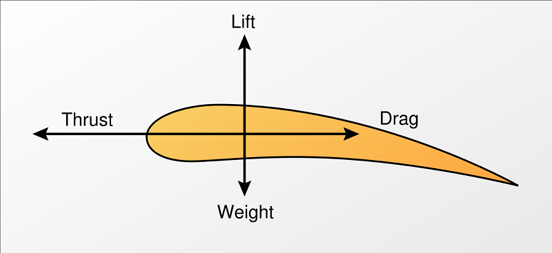Forces on an aerofoil

A fixed-wing aircraft generates forward thrust when air is pushed in the direction opposite to flight. This can be done in several ways including by the spinning blades of a propeller, or a rotating turbine pushing air from the back of a jet engine, or by ejecting hot gases from a rocket engine. The forward thrust is proportional to the mass of the airstream multiplied by the velocity of the airstream. Reverse thrust can be generated to aid braking after landing by reversing the pitch of variable pitch propeller blades, or using a thrust reverser on a jet engine. Rotary wing aircraft and thrust vectoring V/STOL aircraft use engine thrust to support the weight of the aircraft, and vector some of this thrust fore and aft to control forward speed.

Birds normally achieve thrust during flight by flapping their wings.

A motorboat generates thrust (or reverse thrust) when the propellers are turned to accelerate water backwards (or forwards). The resulting thrust pushes the boat in the opposite direction to the sum of the momentum change in the water flowing through the propeller.

A rocket is propelled forward by a thrust force equal in magnitude, but opposite in direction, to the time-rate of momentum change of the exhaust gas accelerated from the combustion chamber through the rocket engine nozzle. This is the exhaust velocity with respect to the rocket, times the time-rate at which the mass is expelled, or in mathematical terms: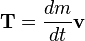$\mathbf{T}=\frac{dm}{dt}\mathbf{v}$

where:

• T is the thrust generated (force)
•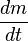$\frac {dm} {dt}$ is the rate of change of mass with respect to time (mass flow rate of exhaust);
• v is the speed of the exhaust gases measured relative to the rocket.

For vertical launch of a rocket the initial thrust must be more than the weight.

Each of the three Space shuttle main engines can produce a thrust of 1.8 MN, and each of its two Solid Rocket Boosters 14.7 MN, together 29.4 MN. Compare with the mass at lift-off of 2,040,000 kg, hence a weight of 20 MN.

By contrast, the simplified Aid for EVA Rescue (SAFER) has 24 thrusters of 3.56 N each.

In the air-breathing category, the AMT-USA AT-180 jet engine developed for radio-controlled aircraft produce 90 N (20 lbf) of thrust. The GE90-115B engine fitted on the Boeing 777-300ER, recognized by the Guinness Book of World Records as the "World's Most Powerful Commercial Jet Engine," has a thrust of 569 kN (127,900 lbf).

## Thrust to power

Thrust at zero speed is zero power. Power requires work to be done, so zero velocity indicates zero work and zero power. Therefore the power of a rocket or aircraft engine is thrust times forward speed.

power (watts) = thrust (newtons) x speed (metres/second)

power (horsepower) = thrust (lbf) x speed (feet/second) / 550

power (horsepower) = thrust (lbf) x speed (feet/minute) / 33000.

For example: the Messerschmitt Me 262 with 3,960 pounds of thrust at 559 mph equates to 5,903 horsepower.

## Thrust to power

A very common question is how to compare the thrust rating of a jet engine with the power rating of a piston engine. Such comparison is difficult, as these quantities are not equivalent. Power is the Force it takes to move something over some Distance divided by the Time it takes to move that distance: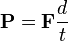$\mathbf{P}=\mathbf{F}\frac{d}{t}$

In case of a rocket or a jet aircraft, the Force is exactly the Thrust produced by the engine. If the rocket or aircraft is moving at about a constant speed, then Distance divided by Time is just Speed, so Power is Thrust times Speed: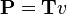$\mathbf{P}=\mathbf{T}{v}$

This formula looks surprising, but it is correct: if a jet aircraft is at full throttle but is tied to a very strong chain to a tree, then mathematically the jet engine will produce no power. It produces a lot of thrust, but it doesn't move, so it performs no work and therefore has zero power.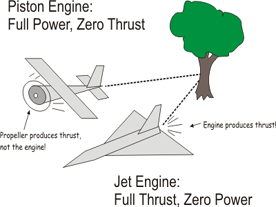Two aircraft tied to a tree

Conversely, a piston engine produces work by turning the propeller, that is, by creating torque on the propeller. It doesn't create any force on the plane by itself; so a piston engine has no thrust. The propeller is the entity that accelerates the air and creates a force in the airframe -- so the propeller is the entity that produces thrust. If you tie a piston aircraft tied to a very strong chain at full throttle, then its engine will have zero thrust and full power.

Now, imagine the strong chain is broken, and the jet and the piston aircraft start to move. At low speeds:

The piston engine will have constant 100% power, and the propeller's thrust will vary with speed
The jet engine will have constant 100% thrust, and the engine's power will vary with speed

One could imagine "okay, so we just multiply the engine thrust by the maximum speed, and we have the equivalent power". That would be true if the actual thrust at full speed was the maximum thrust. But, the thrust changes with speed, altitude and external temperature. In order to provide a single number for publication, engine builders publish the Maximum Static Thrust, that is, the force measured when you put the engine in a test stand in the ground and submit it to full throttle. The actual thrust at speed will be quite different. Additionally, it is unusual to operate jet engines at full throttle continuously. For example, the SR-71 Blackbird requires full throttle to break the sound barrier, but once it reaches Mach 3 the throttle is cut down to 30%.

That way, comparison between Power and Thrust is difficult, as it requires data that is usually not available. One of the cases where it is available is through NASA, which reported that a 747-200 at 40,000 feet requires a calculated thrust of 55,145 lb (25% of its maximum static thrust) to cruise at Mach 0.9. With the equation above we find that is equivalent to power of about 87,000 hp (64880 kW). However, the same aircraft flying at lower altitude than 40,000 feet with the same conditions will have a lower true airspeed, and will be rated at a much lower power.

Published in July 2009.